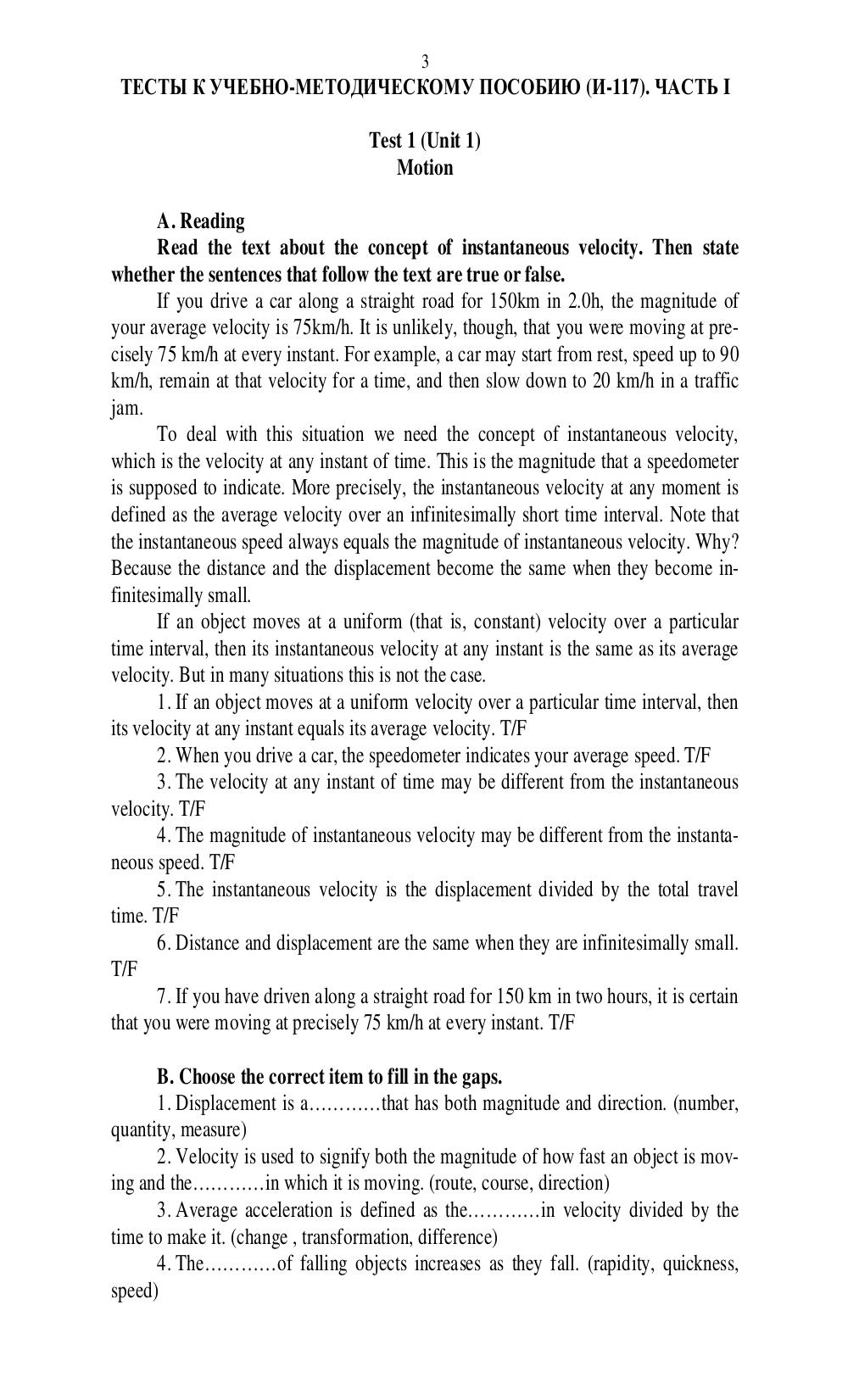# Английский язык. Ч. 1. Ильичева Н.А - 3 стр.

Составители:

Рубрика:

• ## Иностранный язык

3
ТЕСТЫ К УЧЕБНО-МЕТОДИЧЕСКОМУ ПОСОБИЮ (И -117). ЧАСТЬ I
Test 1 (Unit 1)
Motion
whether the sentences that follow the text are true or false.
If you drive a car along a straight road for 150km in 2.0h, the magnitude of
your average velocity is 75km/h. It is unlikely, though, that you were moving at pre-
cisely 75 km/h at every instant. For example, a car may start from rest, speed up to 90
km/h, remain at that velocity for a time, and then slow down to 20 km/h in a traffic
jam.
To deal with this situation we need the concept of instantaneous velocity,
which is the velocity at any instant of time. This is the magnitude that a speedometer
is supposed to indicate. More precisely, the instantaneous velocity at any moment is
defined as the average velocity over an infinitesimally short time interval. Note that
the instantaneous speed always equals the magnitude of instantaneous velocity. Why?
Because the distance and the displacement become the same when they become in-
finitesimally small.
If an object moves at a uniform (that is, constant) velocity over a particular
time interval, then its instantaneous velocity at any instant is the same as its average
velocity. But in many situations this is not the case.
1. If an object moves at a uniform velocity over a particular time interval, then
its velocity at any instant equals its average velocity. T/F
2. When you drive a car, the speedometer indicates your average speed. T/F
3. The velocity at any instant of time may be different from the instantaneous
velocity. T/F
4. The magnitude of instantaneous velocity may be different from the instanta-
neous speed. T/F
5. The instantaneous velocity is the displacement divided by the total travel
time. T/F
6. Distance and displacement are the same when they are infinitesimally small.
T/F
7. If you have driven along a straight road for 150 km in two hours, it is certain
that you were moving at precisely 75 km/h at every instant. T/F
B. Choose the correct item to fill in the gaps.
1. Displacement is a……that has both magnitude and direction. (number,
quantity, measure)
2. Velocity is used to signify both the magnitude of how fast an object is mov-
ing and the…………in which it is moving. (route, course, direction)
3. Average acceleration is defined as the……in velocity divided by the
time to make it. (change , transformation, difference)
4. The…………of falling objects increases as they fall. (rapidity, quickness,
speed)3
ТЕСТЫ К УЧЕБНО-МЕТОДИЧЕСКОМУ ПОСОБИЮ (И-117). ЧАСТЬ I

Test 1 (Unit 1)
Motion

whether the sentences that follow the text are true or false.
If you drive a car along a straight road for 150km in 2.0h, the magnitude of
your average velocity is 75km/h. It is unlikely, though, that you were moving at pre-
cisely 75 km/h at every instant. For example, a car may start from rest, speed up to 90
km/h, remain at that velocity for a time, and then slow down to 20 km/h in a traffic
jam.
To deal with this situation we need the concept of instantaneous velocity,
which is the velocity at any instant of time. This is the magnitude that a speedometer
is supposed to indicate. More precisely, the instantaneous velocity at any moment is
defined as the average velocity over an infinitesimally short time interval. Note that
the instantaneous speed always equals the magnitude of instantaneous velocity. Why?
Because the distance and the displacement become the same when they become in-
finitesimally small.
If an object moves at a uniform (that is, constant) velocity over a particular
time interval, then its instantaneous velocity at any instant is the same as its average
velocity. But in many situations this is not the case.
1. If an object moves at a uniform velocity over a particular time interval, then
its velocity at any instant equals its average velocity. T/F
2. When you drive a car, the speedometer indicates your average speed. T/F
3. The velocity at any instant of time may be different from the instantaneous
velocity. T/F
4. The magnitude of instantaneous velocity may be different from the instanta-
neous speed. T/F
5. The instantaneous velocity is the displacement divided by the total travel
time. T/F
6. Distance and displacement are the same when they are infinitesimally small.
T/F
7. If you have driven along a straight road for 150 km in two hours, it is certain
that you were moving at precisely 75 km/h at every instant. T/F

B. Choose the correct item to fill in the gaps.
1. Displacement is a…………that has both magnitude and direction. (number,
quantity, measure)
2. Velocity is used to signify both the magnitude of how fast an object is mov-
ing and the…………in which it is moving. (route, course, direction)
3. Average acceleration is defined as the…………in velocity divided by the
time to make it. (change , transformation, difference)
4. The…………of falling objects increases as they fall. (rapidity, quickness,
speed)